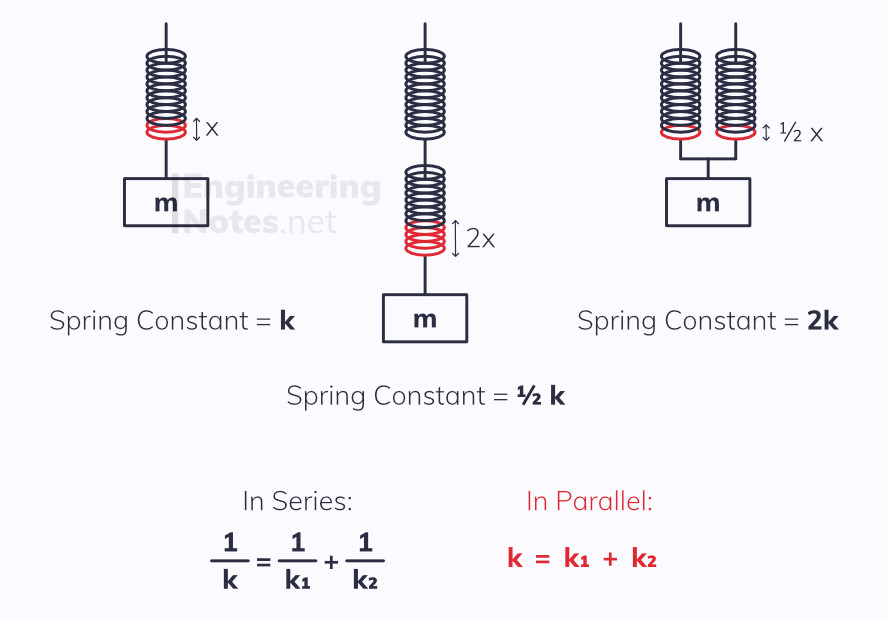#### Notes by Category University Engineering

Electronics*
Mathematics*
Mechanics & Stress Analysis*
Rate these notesNot a fanNot so goodGoodVery goodBrillRate these notes
• A-Level Physics

# Materials

Materials can have many different properties, and experience a number of external and internal forces. The two main such forces are the tensile and compressive forces, relating to extension (stretching) and compression (squishing) respectively.

## Hooke's Law

The most common example of these forces being applied are in a helical spring: these have a natural length, and are either stretched or compressed depending on direction of force applied. The distance of this deformation is called the extension (regardless of direction) and according to Hooke's law:

Extension is directly proportional to the force applied, provided the elastic limit is not passedAs you can see in the graph, the relationship is linear, where the gradient is the force constant up to the elastic limit - if this is exceeded, the object will no longer return to its initial shape, - plastic deformation has occurred.

F = kx Force = force constant x extension

### Multiple String Systems

Springs (and indeed any elastic materials) can be combined either in series or in parallel, with vastly different effects:## Elastic Potential Energy

All springs, just like any object under tensile or compressive forces, store Elastic Potential Energy. This is equivalent to the work done in extending/compressing them, and since W = Fx, this is the area under a force-extension graph.W = 1/2 Fx E = 1/2 Fx E = 1/2 k x^2